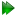Subsections

Double pivoted motions

When the motion of a multiple rigid body system can be described as two rotations about two different pivots, the modulation of the PCS becomes more complicated. Figure 12.1 shows this motion for a single lanthanide to atom vector.Atomic level mechanics of the double pivot

The six vectors at the original position areThe six vectors after the first rotation for state i, Ri(1), areThe six vectors after the second rotation for state i, Ri(2), arePCS and double pivoted motions

As defined in equation 12.59 on page, the PCS for state i is

 δ =riT⋅A⋅ri. (12.64)

For the double motion of the lanthanide-atom vector rLN(2), this becomes